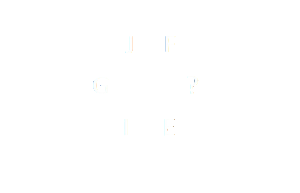Home > Math Shortcuts > Brain Trigger #31 – Sample Letters puzzles question

# Brain Trigger #31 – Sample Letters puzzles question

Questions on Letters puzzles are very common in competitive exams and here in this section we will discuss few of them with answer. These types of Letters puzzles questions will help you to prepare for your exam. To solve these questions you need to apply your math skills. Apply your skills on shortcut tricks to solve these Letters puzzles question.

We try to bring together all types of shortcut methods on Letters puzzles for every topic here in this website. what you need to do is to apply these tricks in Letters puzzles problem to solve. However it’s possible that you may not need any tricks to solve few questions.

We will discuss here a question on Letters puzzles. Read the provided question on Letters puzzles very carefully and try your best to solve the question. We provide the answer below. If you do it yourself then check your answer with our solution. You can also check below if you don’t know how to do the problem correctly.

Each page is having a question on Letters puzzles and also contain its solution with detail explanation. Check other questions by clicking on the Next/Previous link below.

Let’s begin the question and answer session. Today’s question is:

What should be the letter replaces in the question mark ?To know the solution of this problem please scroll down below. You can take as much time as you want to answer the problem. But try to solve this question as quickly as you can.

(Scroll down to get the answer)

(Scroll down to get the answer)

(Scroll down to get the answer)

(Scroll down to get the answer)

Here starts the solution of the above problem. If you solve the question and got an answer then check your answer with our answer. A very Well Done if your answer is correct, but don’t be panic if you are unable to answer the question correctly. Solution with explanation of the following question is provided below.

You can do in this way…..

sum of position of each letter in opposite equal to fifteen
J + E = 10 + 5 = 15
G + H = 7 + 8 = 15
I + F = 9 + 6 = 15